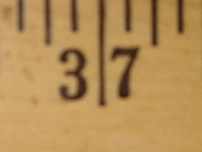# 37

This number is a prime.

Just showing those entries submitted by 'Gaydos': (Click here to show all)There is a total of 37 decimal digits in the Collatz trajectory for 37. [Gaydos]37! is the smallest factorial number divisible by 666 that produces a beastly number when divided by 666. [Gaydos]The smallest positive integer that is never prime when followed by a string of ones, i.e., 371, 3711, 37111, 371111, ... are all composite numbers for any number of ones. [Gaydos]37 distinct digit positive integers have distinct digit cubes. [Gaydos]The most common sum of digits of the distinct-digit primes is 37. [Gaydos]The most common sum of digits of the distinct-digit semiprimes is 37. [Gaydos]37 distinct-digit numbers have distinct-digit cubes. [Gaydos]

(There are 2 curios for this number that have not yet been approved by an editor.)